#The Method of Partial Fractions

## Introduction

Early in Algebra you learn how to combine "simple'' fractions into a "more complicated'' one. Here is a typical example: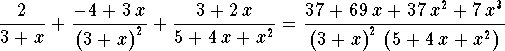The Method of Partial Fractions does the opposite: It dissects a complicated fraction into a sum of simple fractions. While this is a little more complicated than going the other direction, it is also more useful. Major applications of the method of partial fractions include:

## How simple can it get?

A simple fraction is a fraction with a simple denominator. The first step consists of detecting the factors (the building blocks) of the given denominator. The Fundamental Theorem of Algebra tells us what is possible: Every polynomial can be factored into linear factors (degree 1 polynomials) and irreducible polynomials of degree 2.

#### Some Examples.

• The polynomial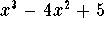can be factored into three linear factors as follows (Do you remember how to do this?):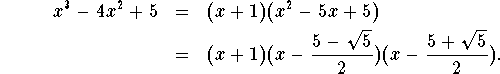• The polynomial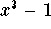has a linear factor and an irreducible factor of degree 2: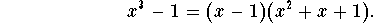• The polynomial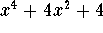has a twice repeated irreducible factor of degree 2: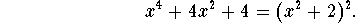#### How can you tell whether a degree 2 polynomial is irreducible (over the field of real numbers), or can be factored further into two linear factors?

There are different methods to decide:

• Graphically: A reducible quadratic polynomial has 2 zeros or one repeated zero, an irreducible quadratic polynomial has no zeros!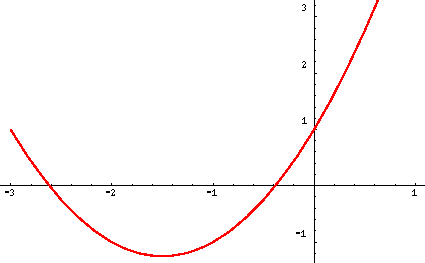This quadratic polynomial can be factored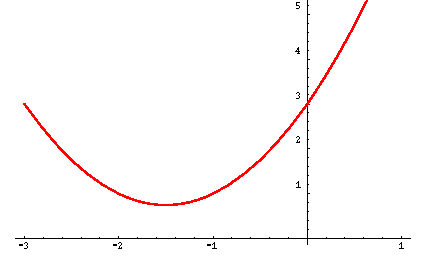An irreducible quadratic polynomial

• Algebraically: If the quadratic formula results in a negative expression under the radical (the discriminant), the associated polynomial is irreducible: Consider the polynomial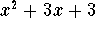: Using the quadratic formula for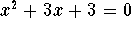yields: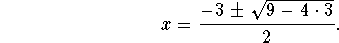Since the discriminant (the expression under the radical) is negative, the polynomial is irreducible!

#### Try it yourself!

Is the polynomial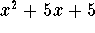irreducible or reducible?

Helmut Knaust
Fri Jul 5 13:54:22 MDT 1996